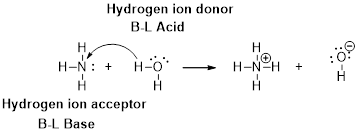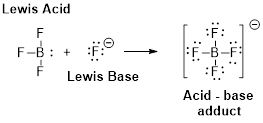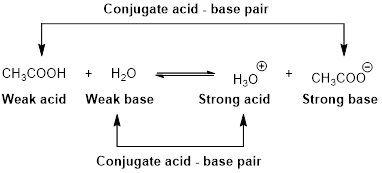Chapter 16, Problem 2PS

Chapter
Section
Textbook Problem

Write the formula and give the name of the conjugate base of each of the following acids. a) NH 3 b) HCO 3 − c) Br −

(a)

Interpretation Introduction

Interpretation:

Formula and conjugated acid of given base has to be identified.

Concept Introduction:

Arrhenius Acids and Bases

Acid release hydrogen ion in water

Base release hydroxide ions in water.

HCl(aq) H+(aq) + Cl-(aq).........AcidNaOH(aq) Na+(aq)+ OH-(aq)...........Base

An acid is a substance that produces hydronium ions, H3O+ when dissolved in water.

Bronsted –Lowry definitions:

A Bronsted –Lowry acid is a proton donor, it donates a hydrogen ion, H+

A Bronsted-Lowry base is a proton acceptor, it accepts a hydrogen ion H+Lewis definition:

A Lewis acid is a substance that can accept and share an electron pair.

A Lewis base is a substance that can donate and share an electron pair.Bronsted –Lowry conjugated acid-Base pairs:

When an acid is dissolved in water, the acid (HA) donates a proton to water to form a new acid (conjugated acid) and a new base (conjugated base).The pair of an Acid –Base differs by a proton called conjugated Acid-Base pair.

Explanation

NH3 is a base . Ammonium ion (NH4+) is a conjugated acid. NH3 and (

(b)

Interpretation Introduction

Interpretation:

Formula and conjugated acid of given base has to be identified.

Concept Introduction:

Arrhenius Acids and Bases

Acid release hydrogen ion in water

Base release hydroxide ions in water.

HCl(aq) H+(aq) + Cl-(aq).........AcidNaOH(aq) Na+(aq)+ OH-(aq)...........Base

An acid is a substance that produces hydronium ions, H3O+ when dissolved in water.

Bronsted –Lowry definitions:

A Bronsted –Lowry acid is a proton donor, it donates a hydrogen ion, H+

A Bronsted-Lowry base is a proton acceptor, it accepts a hydrogen ion H+Lewis definition:

A Lewis acid is a substance that can accept and share an electron pair.

A Lewis base is a substance that can donate and share an electron pair.Bronsted –Lowry conjugated acid-Base pairs:

When an acid is dissolved in water, the acid (HA) donates a proton to water to form a new acid (conjugated acid) and a new base (conjugated base).The pair of an Acid –Base differs by a proton called conjugated Acid-Base pair.

(c)

Interpretation Introduction

Interpretation:

Formula and conjugated acid of given base has to be identified.

Concept Introduction:

Arrhenius Acids and Bases

Acid release hydrogen ion in water

Base release hydroxide ions in water.

HCl(aq) H+(aq) + Cl-(aq).........AcidNaOH(aq) Na+(aq)+ OH-(aq)...........Base

An acid is a substance that produces hydronium ions, H3O+ when dissolved in water.

Bronsted –Lowry definitions:

A Bronsted –Lowry acid is a proton donor, it donates a hydrogen ion, H+

A Bronsted-Lowry base is a proton acceptor, it accepts a hydrogen ion H+Lewis definition:

A Lewis acid is a substance that can accept and share an electron pair.

A Lewis base is a substance that can donate and share an electron pair.Bronsted –Lowry conjugated acid-Base pairs:

When an acid is dissolved in water, the acid (HA) donates a proton to water to form a new acid (conjugated acid) and a new base (conjugated base).The pair of an Acid –Base differs by a proton called conjugated Acid-Base pair.

Still sussing out bartleby?

Check out a sample textbook solution.

See a sample solution

The Solution to Your Study Problems

Bartleby provides explanations to thousands of textbook problems written by our experts, many with advanced degrees!

Get Started